﻿ 一种机载SAR快速几何精校正算法
«上一篇文章快速检索 高级检索

 雷达学报2016, Vol. 5Issue (4): 419-424  DOI: 10.12000/JR160640

### 引用本文 [复制中英文]

[复制中文]
Liu Yunlong , Li Yanlei , Zhou Liangjiang , Liang Xingdong . A Fast Precise Geometric Calibration Method for Airborne SAR[J]. Journal of Radars, 2016, 5(4): 419-424. DOI: 10.12000/JR16064.
[复制英文]

### 文章历史

(中国科学院大学 北京 100049)
(中国科学院电子学研究所微波成像技术国家级重点实验室 北京 100190)

A Fast Precise Geometric Calibration Method for Airborne SAR
(University of Chinese Academy of Sciences, Beijing 100049, China)
(National Key Laboratory of Science and Technology on Microwave Imaging, Institute of Electronics, Chinese Academy of Sciences, Beijing 100190, China)
Foundation Item: The National 863 Program of China (2013AA092105), The Public Welfare Special Project for Surveying and Mapping (201412002)
Abstract: Geometric positioning accuracy is an important technical indicator for Synthetic Aperture Radar (SAR) in the geomatics domain. Airborne SAR is an important trend in SAR technology due to its advantages of high flexibility, high resolution, and low cost. Motion error and undulating terrain are the major sources of airborne SAR geometric positioning. In this paper, we consider the SAR imaging principle and imaging geometry to carefully examine the mechanism of geometric positioning error caused by the coupling of motion error and terrain fluctuation. Therefore, we propose a fast geometric precision correction method and verify the validity and effectiveness of the proposed method based on simulation results and experimental data.
Key words: Airborne Synthetic Aperture Radar (SAR)    Geometric calibration    Residual phase error    Motion Compensation (MoCo)    Motion error    Terrain fluctuation
1 引言

2 算法原理

 $\Delta x = - {r_0}\frac{{\partial \delta \Delta r}}{{\partial x}}$ (1)

 $\Delta x=-{{r}_{0}}\frac{\delta \Delta {{r}_{L}}}{L}=-\frac{\delta \Delta {{r}_{L}}}{2\tan ({{\theta }_{\text{bw}}}/2)}$ (2)

2.1 平地假设和波束中心近似导致的相位误差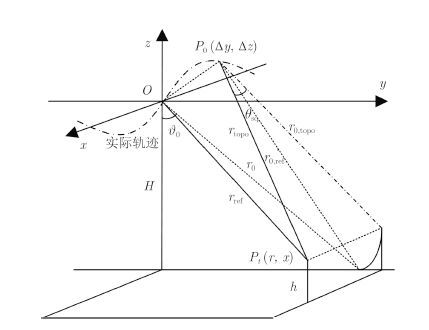图 1 运动补偿几何关系 Fig.1 Geometry of motion compensation

 \begin{align} & {{\phi }_{\text{ref}}}(r,x)=\frac{4\pi }{\lambda }\Delta {{r}_{0,\text{ref}}}(r,x)= \\ & \frac{4\pi }{\lambda }({{r}_{0,\text{ref}}}(r,x)-{{r}_{0}}(r,x)) \\ \end{align} (3)

 \begin{aligned} {\phi _{{\rm{topo}}}}(r,x) = & \frac{4\pi }{\lambda }\Delta {r_{{\rm{topo}}}}(r,x)\\ = & \frac{4\pi }{\lambda }({r_{{\rm{topo}}}}(r,x) - {r_{{\rm{ref}}}}(r,x)) \end{aligned} (4)

 \begin{aligned} \Delta {r_{{\rm{topo}}}}(r,x) = & {r_0}\sqrt {{{\bigg(\frac{{\Delta {r_{0,{\rm{topo}}}}}}{{{r_0}}} + 1\bigg)}^2} + {{\tan }^2}{\theta _{{\rm{sq}}}}(x)} \\ & - {r_0}\sqrt {1 + {{\tan }^2}{\theta _{{\rm{sq}}}}(x)} \\ \approx & \Delta {r_{0,{\rm{topo}}}} \cdot \cos {\theta _{{\rm{sq}}}}(x) \end{aligned} (5)

 \begin{aligned} {\phi _{{\rm{err,topo}}}}(r,x) = & {\phi _{{\rm{topo}}}}(r,x) - {\phi _{{\rm{ref}}}}(r,x)\\ \approx & \frac{4\pi }{\lambda }({r_{{\rm{0,topo}}}} - {r_{\rm{0}}}) \cdot \cos {\theta _{{\rm{sq}}}}(x)\\ & - \frac{4\pi }{\lambda }({r_{0,\; {\rm{ref}}}} - {r_{\rm{0}}}) \end{aligned} (6)

 ${\phi _{{\rm{err,topo}}}}(r,x) \approx \frac{4\pi }{\lambda }({r_{{\rm{0,topo}}}} - {r_{0,{\rm{ref}}}})$ (7)

 ${r_{0,{\rm{ref}}}} \!=\! \sqrt {{{(H + \Delta z)}^2} + {{\Big(\sqrt {r_0^2 - {H^2}} + \Delta y\Big)}^2}}$ (8)
 ${r_{0,{\rm{topo}}}} \!=\! \sqrt {{{(H \!-\! h \!+\! \Delta z)}^2} \!+\! {{\Big(\sqrt {r_0^2 \!-\! {{(H \!-\! h)}^2}} \!\!+\! \!\Delta y\Big)}^2}}$ (9)
2.2 方位向快速几何精校正

2.1小节分析了平地假设和波束中心近似导致的相位误差，并通过推导获得了相位误差的表达式Φerr,topo(r,x)。由于方位向几何定位误差与残余运动误差导致的残余相位误差线性项成正比[3, 4]，可通过计算Φerr,topo(r,x)的线性项系数来校正方位向几何定位误差。

 \begin{aligned} \frac{{d{\phi _{{\rm{err,topo}}}}}}{{dx}} = & \frac{{\partial \phi }}{{\partial \Delta y}}\frac{{d\Delta y}}{{dx}} + \frac{{\partial \phi }}{{\partial \Delta z}}\frac{{d\Delta z}}{{dx}}\\ \approx & \frac{{4{{π}} \Big(\sqrt {r_0^2 - {{(H - h)}^2}} - \sqrt {r_0^2 - {H^2}} \Big)}} {{\lambda {r_0}}}\\ & \cdot \frac{{d\Delta y}}{{dx}} - \frac{{4{{π}} h}}{{\lambda {r_0}}}\frac{{d\Delta z}}{{dx}} \end{aligned} (10)

 $\Delta x=-\frac{1}{2\pi }\frac{d{{\phi }_{\text{err},\text{topo}}}}{dx}$ (11)

 $\left. \begin{gathered} \sum {{\text{ }}y_i^{{\text{cur}}}} = \sum {{\text{ }}y_i^{{\text{pre}}}} - {\text{ }}y_1^{{\text{pre}}} + {\text{ }}y_N^{{\text{cur}}} \hfill \\ \sum {x_i}{y_i}^{{\text{cur}}} = \sum {{x_i}{\text{ }}y_i^{{\text{pre}}}} - {x_1}{\text{ }}y_1^{{\text{pre}}} + {x_N}{y_N}^{{\text{cur}}} \hfill \\ \end{gathered} \right\}$ (13)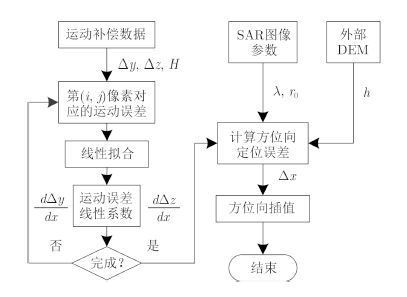图 2 算法流程 Fig.2 Algorithm flow
2.3 快速算法运算量分析表 1 PTA算法和快速算法运算量比较 Tab.1 PTA and fast algorithm operation quantity comparison
3 仿真分析表 2 仿真参数 Tab.2 Simulation parameters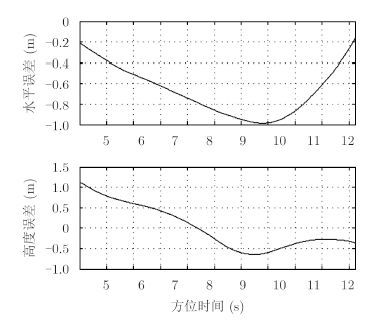图 3 Δy和Δz历程 Fig.3 Histories of Δy and Δz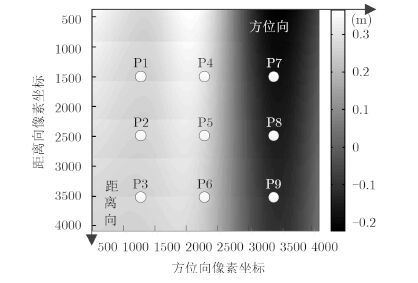图 4 点目标布置和Δx分布 Fig.4 Target locations and distribution of Δx表 3 仿真结果 Tab.3 The result of simulation

4 实测数据验证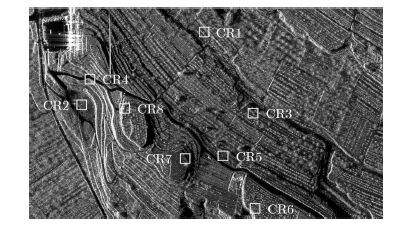图 5 成像结果和定标点位置 Fig.5 Imaging result and position of the calibration points表 4 实测数据结果 Tab.4 The result of measured data

SAR图像尺寸为方位向4096像素点距离向8192像素点。分别使用PTA算法和本文提出的快速算法对SLC进行几何校正处理，PTA算法耗时53 min 20 s，快速算法耗时9 min 28 s，效率提高4.6倍，再次验证了快速算法的效率。经过两种算法处理后，方位向几何定位残余误差见表 4

5 结论

  苗慧. 机载SAR定位精度的研究[D]. [博士论文], 中国科学院电子学研究所, 2007. iao Hui. Research on airborne SAR geolocation accuracy[D]. [Ph.D. dissertation], Institute of Electronics, Chinese Academy of Sciences, 2007. (0)  Buckreuss S. Motion errors in an airborne synthetic aperture radar system[J]. European Transactions on Telecommunications,1991, 2 (6) : 655 –664. (0)  Fornaro G, Franceschetti G, Perna S. Motion compensation errors: effects on the accuracy of airborne SAR images[J]. IEEE Transactions on Aerospace and Electronic Systems,2005, 41 (4) : 1338 –1352. (0)  Blacknell D, Freeman A, Quegan S, et al. Geometric accuracy in airborne SAR images[J]. IEEE Transactions on Aerospace and Electronic Systems,1989, 25 (2) : 241 –258. (0)  李银伟, 韦立登, 向茂生. 机载干涉SAR运动补偿中地物目标定位误差的影响分析[J]. 雷达学报,2013, 2 (4) : 492 –498. Li Yin-wei, Wei Li-deng, Xiang Mao-sheng. Effects of target positioning error on motion compensation for airborne interferometric SAR[J]. Journal of Radars,2013, 2 (4) : 492 –498. (0)  Moreira A, Huang Y. Airborne SAR processing of highly squinted data using a chirp scaling approach with integrated motion compensation[J]. IEEE Transactions on Geoscience and Remote Sensing,1994, 32 (5) : 1029 –1040. (0)  Fornaro G, Franceschetti G, Perna S. On center-beam approximation in SAR motion compensation[J]. IEEE Geoscience and Remote Sensing Letters,2006, 3 (2) : 276 –280. (0)  李银伟, 邓袁, 向茂生. 波束中心近似对机载干涉SAR运动补偿的影响分析[J]. 电子与信息学报,2014, 36 (2) : 415 –421. Li Yin-wei, Deng Yuan, Xiang Mao-sheng. Effects of center-beam approximation on motion compensation for airborne interferometric SAR[J]. Journal of Electronics & Information Technology,2014, 36 (2) : 415 –421. (0)  韦立登, 向茂生, 吴一戎. POS数据在机载干涉SAR运动补偿中的应用[J]. 遥感技术与应用,2007, 22 (2) : 188 –194. Wei Li-deng, Xiang Mao-sheng, Wu Yi-rong. Application of POS data in airborne interferometric SAR motin compensation[J]. Remote Sensing Technology and Application,2007, 22 (2) : 188 –194. (0)  Prats P, Reigber A, Mallorqui J. Topography-dependent motion compensation for repeat-pass interferometric SAR systems[J]. IEEE Geoscience and Remote Sensing Letters,2005, 2 (2) : 206 –210. (0)  唐晓青, 向茂生, 吴一戎. 一种改进的基于DEM的机载重轨干涉SAR运动补偿算法[J]. 电子与信息学报,2009, 31 (5) : 1090 –1094. Tang Xiao-qing, Xiang Mao-sheng, Wu Yi-rong. An improved topography-dependent motion compensation approach for airborne repeat-pass interferometric SAR systems[J]. Journal of Electronics & Information Technology,2009, 31 (5) : 1090 –1094. (0)  Potsis A, Reigber A, Mittermayer J, et al. Sub-aperture algorithm for motion compensation improvement in wide-beam SAR data processing[J]. Electronics Letters,2001, 37 (23) : 1405 –1407. (0)  heng X, Yu W, and Li Z. A novel algorithm for wide beam SAR motion compensation based on frequency division methods[C]. IEEE Geoscience and Remote Sensing Symposium (IGARSS), Denver, CO, USA, 2006: 3160-3163. (0)  De Macedo K A C, Scheiber R. Precise topography- and aperture-dependent motion compensation for airborne SAR[J]. IEEE Geoscience and Remote Sensing Letters,2005, 2 (2) : 172 –176. (0)